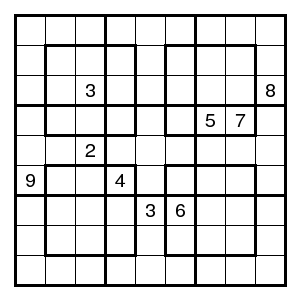# NRC Sudokus

The Dutch newspaper NRC has every Saturday an NRC-Sudoku puzzle. An example from 2005-12-31 (by P. Ritmeester):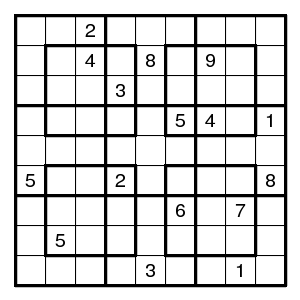One has to complete this grid under the normal Sudoku rules (each digit 1-9 occurs precisely once in every row, column or box), but there are 13 boxes instead of the usual 9.

## More boxes

For an ordinary Sudoku it is enough to require four boxes (and the rest will follow automatically, provided the four boxes do not cover a row or a column, or form the symmetric difference of a row and a column). The same holds here: one also has nine boxes - the four given in advance, and five that follow automatically. In the pictures below the orange box is a complement of two NRC-boxes in the union of three columns. There are four of such boxes (two in the union of three columns, and two in the union of three rows). And the red box is the complement of the 4+4 found so far. These additional boxes are very useful when solving NRC-Sudokus - they show that certain cells must have different digits where this is not immediately apparent.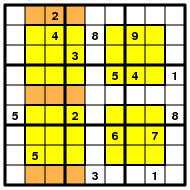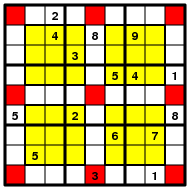## Fewer clues

For ordinary Sudokus the smallest number of clues known (for a puzzle with unique solution) is 17. Since we have stronger restrictions here, fewer clues are needed. About 5% of the puzzles produced by my random NRC-Sudoku generator use 14 clues. An example: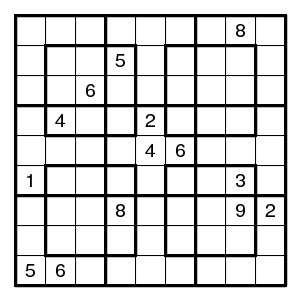About 0.1% uses 13 clues. An example: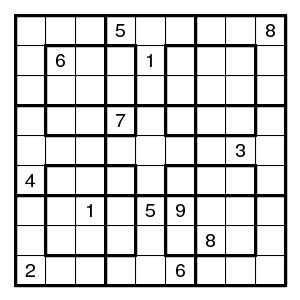Every now and then one encounters examples with 12 clues. A dedicated search yields thousands of them. An example: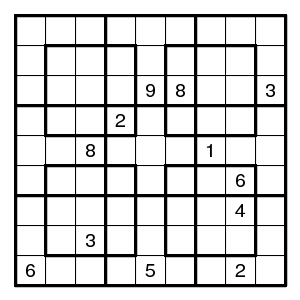A dedicated search yielded a few examples with 11 clues.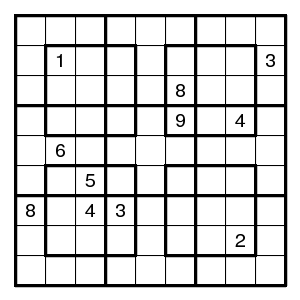## Counting

The total number of completed NRC Sudokus is 6337174388428800.

(Found by aeb, confirmed by Red Ed ("T 145").)

## Also requiring the diagonals

A diagonal NRC Sudoku, short NRCX Sudoku, is one where also both diagonals are permutations. Here two examples with 9 clues.